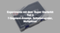This article is about the display of digits with 7-segment displays. For the control, a microcontroller is used. All segments are controlled directly or via a shift register. For the control of a display with four digits, the multiplex technique is used.

Required hardware

 Number Component Note 1 Super Starter Kit 1 9V block battery or 9V power supply unit optional

7-segment display 5161AS

A 7-segment display consists of eight LEDs. One for each segment and one for a decimal point. To keep the number of connections small, all cathodes or anodes are combined and led out at one pin. The display in the starter kit has a common cathode. The following figures show the display with the designation of the segments and the pin assignment, as well as the internal circuit.Connection to the microcontroller and test program

The easiest way is to connect the anode of each light-emitting diode to an output of the microcontroller. Again, as with the simple light-emitting diode, it is necessary to preconnect a resistor to limit the current. Resistors of 1 kΩ can be used, as shown in Part 2. I chose 4.7 kΩ, because in a later example also the cathode shall be controlled by an output of the microcontroller. When all diodes are lit, a total current of 3 V / 4700 Ω * 8 = 5.1 mA results at the common cathode. This current can be handled by an output of the microcontroller without any problems. The display is hardly darker with 4.7 kΩ than with 1 kΩ.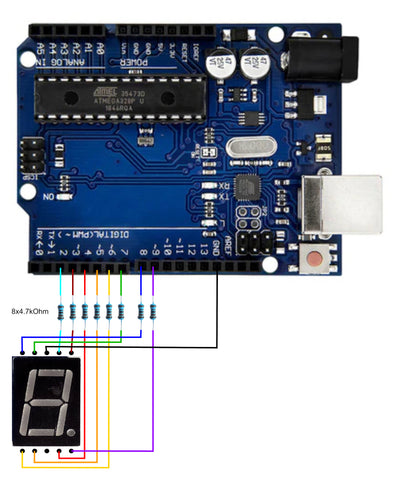Since in the program the content of a byte must be decomposed into individual bits, logical operators are used, which link to the individual bits of a byte. The following operators are available:
Logical AND operation c = a & b
a 10011011
b 01010101
c 00010001
In the result, all bits are defined in a and b sets

Logical OR operation c = a | b
a 10011011
b 01010101
c 11010111
As a result, all bits are specified in a or b sets

Logical EXCLUSIVE OR operation c = a ^ b
a 10011011
b 01010101
c 11001110
As a result, sets that are specified in a and b are different

Shift to the right c = a >> 2
a 10011010
c 00100110
As a result, all bits are shifted to the right by the number of bits specified in the second operand. The right bits are lost. Zeros are shifted from the left.

Shift to the left c = a << 2
a 10011010
c 01101000
As a result, all bits are shifted to the left by the number of bits specified in the second operand. The left bits are lost. Zeros are shifted from the right.
All logical operators work not only for bytes but also for 16-bit and 32-bit integers.

The program starts again with the definition of the used pins.

#define PINA 2

It is enough to define the pin for segment a because the other pins are used one after the other. So that digits can be displayed, a table is needed from which the information can be taken, and which segments must light up for a certain number.
Each segment is assigned a bit of a byte. So segment a = bit 0, segment b = bit 1, and so on.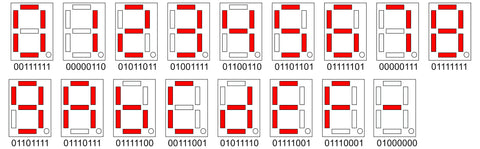byte segments = {
// hgfedcba
0B00111111, //0
0B00000110, //1
0B01011011, //2
0B01001111, //3
0B01100110, //4
0B01101101, //5
0B01111101, //6
0B00000111, //7
0B01111111, //8
0B01101111, //9
0B01110111, //A
0B01111100, //b
0B00111001, //C
0B01011110, //d
0B01111001, //E
0B01110001, //F
0B01000000, //-
};

This is followed by a global variable that determines whether the decimal point should be lit or not.

boolean pkt;

For the display of a digit, a separate function is to be used this time. The advantage of an own function is the encapsulation of a certain task. The declaration starts with the data type that the function returns after its execution, or voidif nothing is returned. This is followed by the name of the function and, in parentheses, a listing of variables that are passed to the function as parameters. The program code for the function follows in looped brackets.

void print7(byte number, boolean dot) {
byte bits;
if (number < 17) {
bits = segments[number];
for (byte i = 0; i < 7; i++) {
digitalWrite(PINA+i, bits & 1);
bits = bits >> 1;
}
digitalWrite(PINA + 7, dot);
}
}

The function is called print7and does not return any data. As the parameter receives the number to the output number, the Boolean variable point controls whether the point is displayed or not. In the function a local variable</7;> bits is defined, which is used as a buffer for the output of the bit pattern. The condition if (number < 17) ensures that the number occurs in the table of bit patterns. The table contains 17-bit patterns with indices 0 to 16. With the parameter number as an index, the corresponding bit pattern is copied from the table into the variable bits variable. Now the segments must be switched on or off one after the other according to the bit pattern. For this purpose, the command for is used, which defines an iteration loop. The keyword is followed by the loop condition in parentheses. This consists of three parts separated by semicolons. The first part assigns the initial value to the loop variable. The second part contains a condition that must be met for a further run. The third part specifies how the value of the loop variable changes after each pass. This is followed by the program code to be executed within the loop in looped brackets. With the command for (byte i = 0; i < 7; i++) the loop variable i of type byte receives the values 0 to 6 and is incremented by one after each pass. With digitalWrite(PINA+i, bits & 1); a segment is switched on or off. The number of the pin is formed from the number of the first pin plus the loop variable. To get the state for the corresponding segment, the least significant bit of the bit pattern is masked with the logical AND operation bits & 1. Afterwards, the bit pattern is masked with the command bits = bits >> 1; the program code is shifted one bit to the right. This places the second bit in the least significant position. This is repeated until seven of the eight bits of the bit pattern have been processed, ending the loop. A separate treatment is done for the point. For this simply the parameter point is output to the corresponding pin.

void setup() {
for (byte i = 0; i < 8; i++) pinMode(PINA + i, OUTPUT);
pkt = false;
}

In the setup() function all eight pins for the segments are defined as output via a loop. The global variable pkt is set to false set.

void loop() {
for (byte num = 0; num < 17; num++) {
print7(num,pkt);
delay (500);
}
pkt = ! pkt;
}

In the loop() function, another loop is used to call the print7() is called with the numbers 0 to 16, whereby the respective number is displayed. A pause of half a second is inserted between the numbers so that the number can also be read. After all numbers have been displayed once, the state of the variable pkt is reversed so that the decimal point is alternately on or off.

Shift register 74HC595

A shift register consists of several memory cells, each with the output of one memory cell connected to the input of the next. With each clock pulse on the clock line, the information is transferred from the input to the output. The data is therefore shifted by one step with each clock pulse.</7;>The 74HC595 device contains an eight-stage shift register. In addition, it also has an eight-bit memory register, so that the data can be held while new data is shifted into the shift register. The outputs of the memory register are led out on pins. These outputs can be switched on or off by the input OE "Output Enable". Low at this input enables the outputs, and High sets them to high impedance, so that several such devices could be connected in parallel. Low at input SRCLR "Shift Register Clear" sets the contents of the shift register to zero. The input SRClk "Shift Register Clock" is for the clock for shifting. Input RClk "Register Clock" is for the clock to accept the shift register data into the memory register. SER is the input for serial data. QH' is the output for serial data, in case several such blocks are to be connected in series.Control of the display via a shift register 74HC595

By using the shift register, the number of necessary microcontroller outputs can be reduced from eight to three. The segments of the 7-segment display are connected to the outputs of the shift register. The microcontroller provides the serial data on one output. On a second output, it generates the shift clock and on the third the clock for transfer to the memory register.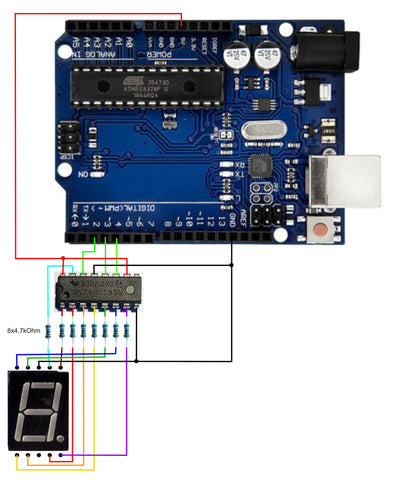In the program for the new circuit, only the function print7() has to be changed. In the beginning, the used pins are defined again.

#define SER 2
#define RCLK 3
#define SRCLK 4

This time the pins for the serial data, for the register clock and for the shift register clock. The table with the bit patterns and the global variable for the dot follows.

byte segmente = {
// hgfedcba
0B00111111, //0
0B00000110, //1
0B01011011, //2
0B01001111, //3
0B01100110, //4
0B01101101, //5
0B01111101, //6
0B00000111, //7
0B01111111, //8
0B01101111, //9
0B01110111, //A
0B01111100, //b
0B00111001, //C
0B01011110, //d
0B01111001, //E
0B01110001, //F
0B01000000, //-
};

boolean pkt;

The function print7() must be changed completely. The segments are no longer controlled directly. The bit patterns are transferred serially to the shift register. For this, first the pin for the serial data must be set with the corresponding value and then a pulse must be generated on the clock line. When all eight bits of the bit pattern have been transferred to the shift register, a pulse is generated on the register clock line to transfer the data from the shift register to the memory register. Since the OE input is fixed low, the bit pattern is displayed on the 7-segment display. This time the bit pattern must be shifted to the left, because the most significant bit of the bit pattern must be shifted first into the shift register.

void print7(byte number, boolean dot) {
byte bits;
if (number < 17) {
bits = segments[number];
if (dot) bits = bits | 0B10000000;
for (byte i = 0; i < 8; i++) {
digitalWrite(SER, bits & 128);
digitalWrite(SRCLK, 1);
digitalWrite(SRCLK, 0);
bits = bits << 1;
}
digitalWrite(RCLK, 1);
digitalWrite(RCLK, 0);
}
}

At the beginning, a local variable for the bit pattern is created. A security check follows to ensure that the number is smaller than 17. Then the bit pattern for the past number from the table is stored in the local variable. If the parameter point is true, the most significant bit is used with a logical OR operation. bits = bits | 0B10000000;

is set. The bit pattern can now be sent to the shift register. This is done in a loop for i = 0 to 7. The most significant bit of the bit pattern is sent with a logical AND operation bits & 128 and written to the pin for the serial data. The clock pulse for the shift register is generated by first writing high and then low to the output. Finally, the content of bits is shifted one place to the left, so that the next bit comes to the most significant position. After all eight bits have been sent in this way, a pulse is also generated at the connection for the register clock.

void setup() {
pinMode(SER, OUTPUT);
pinMode(RCLK, OUTPUT);
pinMode(SRCLK, OUTPUT);
digitalWrite(RCLK, 0);
digitalWrite(SRCLK, 0);
pkt = false;
}

In the setup() function the used pins are defined as output. Furthermore both clock lines are set to low and the variable pkt is set to False.

void loop() {
for (byte num = 0; num < 17; num++) {
print7(num,pkt);
delay (1000);
}
delay(2000);
pkt = ! pkt;
}

The loop() function remains the same as for direct control.

4-digit 7-segment display 5461AS

This display consists of four simple 7-segment displays. To keep the number of connections small, the anodes of the same segments are connected in parallel. The cathodes of each digit are combined and connected to one pin. Only the digit whose cathode is low is lit. So you have to display one digit at a time for a short time. Due to the inertia of the eyes, all four digits are then visible.Control

The control via the shift register is used again. Additionally, four outputs are needed to set the cathodes of the digit that is currently displayed low. Since in extreme cases all segments of a digit are lit, the total current of all eight LEDs must not overload the output of the microcontroller. With the selected series resistors with a value of 4.7 kΩ, there are no problems.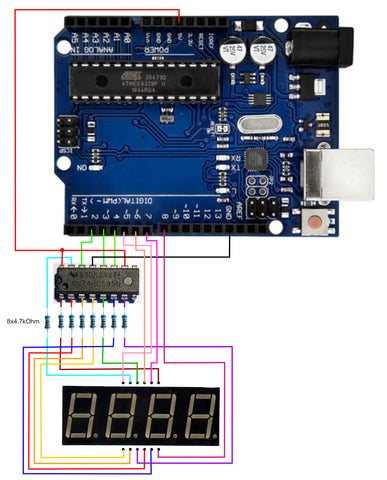Pin 2 is for the serial data, pin 3 for the register clock, and pin 4 for the shift clock. Pin 5 controls the cathodes of the leftmost digit. Pin 6, 7 and 8 control, in order, the other digits.

The test program is to count up and down in the range from -999 to +999 and display the count.

First, all used pins are defined again. The four pins for the cathode control are added.

#define SER 2
#define RCLK 3
#define SRCLK 4
#define DIG1 5
#define DIG2 6
#define DIG3 7
#define DIG4 8

In the table with the bit patterns there is one pattern more, namely all to 0 corresponds to an empty display.

byte segmente = {
// hgfedcba
0B00111111, //0
0B00000110, //1
0B01011011, //2
0B01001111, //3
0B01100110, //4
0B01101101, //5
0B01111101, //6
0B00000111, //7
0B01111111, //8
0B01101111, //9
0B01110111, //A
0B01111100, //b
0B00111001, //C
0B01011110, //d
0B01111001, //E
0B01110001, //F
0B01000000, //-
0B000000, //empty
};

This time there are three global variables. Namely, last a 32-bit unsigned integer number as time memory, number, a signed integer number, and to, a Boolean variable to distinguish between counting up and counting down.

uint32_t last;
int number;
boolean on;

The function print7() is identical to the previous example.

void print7(byte number, boolean dot) {
byte bits;
if (number < 18) {
bits = segments[number];
if (dot) bits = bits | 0B10000000;
for (byte i = 0; i < 8; i++) {
digitalWrite(SER, bits & 128);
digitalWrite(SRCLK, 1);
digitalWrite(SRCLK, 0);
bits = bits << 1;
}
digitalWrite(RCLK, 1);
digitalWrite(RCLK, 0);
}
}

The following is a new function that can display a signed three-digit number.

void displayNum(int Nummer) {
int z;
if ((Nummer >-1000) && (number < 1000)) {
if (number < 0) {
print7(16,false);
number = number * -1;
} else {
print7(17,false);
}
digitalWrite(DIG1,0);
delay(2);
digitalWrite(DIG1,1);
z = number/100;
print7(z,false);</1;>
digitalWrite(DIG2,0);
delay(2);
digitalWrite(DIG2,1);
z = ( number - z * 100) /10;
print7(z,false);
digitalWrite(DIG3,0);
delay(2);
digitalWrite(DIG3,1);
z = number % 10;
print7(z,false);
digitalWrite(DIG4,0);
delay(2);
digitalWrite(DIG4,1);
}
}

In the beginning, a local variable z is defined as an auxiliary memory. The security query follows so that the number passed lies in the permissible range between -999 and 999. The first display is to show the sign. If the number passed is less than 0, the bit pattern with index 16, i.e. the minus sign, is output and the number is multiplied by -1 to obtain a positive number. If the number is positive, the bit pattern is output with index 17, i.e. no segments are lit. With the command sequence

digitalWrite(DIG1,0);
delay(2);
digitalWrite(DIG1,1);

the cathodes of the first 7-segment display are set low for 2 ms so that the segments whose anodes are high are lit.
Now the number is divided by 100. The variable z then contains the hundreds. The variable z is sent to the shift register and then the second digit is switched on for 2 ms. The hundreds are subtracted from the number passed and the remainder is divided by 10. The variable z variable now contains the tens that are output and displayed. Finally, the modulo operator % of the units is determined. These are also output and displayed.

void setup() {
pinMode(SER, OUTPUT);
pinMode(RCLK, OUTPUT);
pinMode(SRCLK, OUTPUT);
pinMode(DIG1, OUTPUT);
pinMode(DIG2, OUTPUT);
pinMode(DIG3, OUTPUT);
pinMode(DIG4, OUTPUT);
digitalWrite(RCLK, 0);
digitalWrite(SRCLK, 0);
digitalWrite(DIG1,1);
digitalWrite(DIG2,1);
digitalWrite(DIG3,1);
digitalWrite(DIG4,1);
number = -99;
up = true;
last = millis();
}

In the setup() function all used pins are defined as outputs. The clock lines are set low and the outputs for the cathodes are set high. The variable number is set to any initial value. Also the variable at for the counting direction can be set arbitrarily. With the assignment last = millis(); the current value of the internal counter is stored in the variable last variable.

void loop() {
displayNum(number);
if ((millis() - last) > 100) {
last = millis();
if (up) {
number++;
if (number > 999) {
number = 998;
to = false;
}
} else {
number--;
if (number < -999) {
number = -998;
up = true;
}
}
}
}

In the loop() function displayNum() is called to display the current counter value. The counter should be changed every 0.1 s. In order to display the current counter value continuously, the delay() function cannot be used here. But you can use the internal counter to find out when the counter should be changed. This is the case when the internal counter is millis() is more than 100 greater than the counter in last is greater than the counter reading stored in last. If this condition is met, the current value of the internal counter for the next interval is stored in the last is stored. Then, depending on the state of the variable to the counter value is increased or decreased. If one of the end values is exceeded, the counting direction is reversed.

Have fun experimenting with the 7-segment displays.